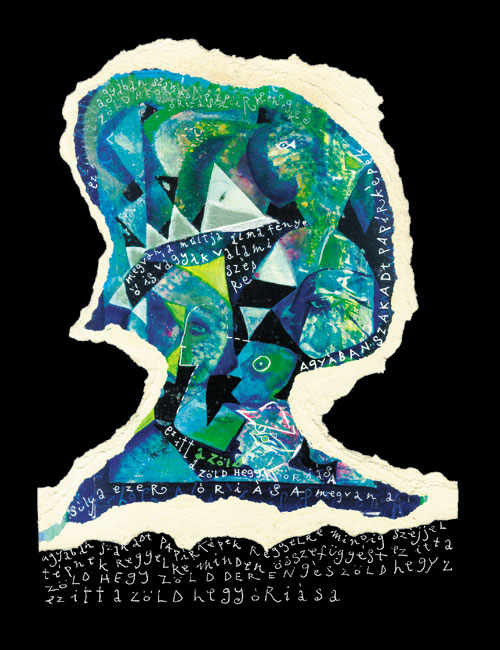A zÃ¶ld hegy Ã³riÃ¡sa

E Z Â I T T Â A Â Z Ã– L D Â H E G Y Â Ã“ R I Ã S A

M E G V A N Â A Â S Ãš L Y A Â E Z E R Â M Ã Z S A

M E G V A N Â A Â M Ãš L T J A Â Ã L M A Â F Ã‰ N Y E

Å Â I S Â V Ã G Y I K Â V A L A M I Â S Z Ã‰ P R E

Å Â I S Â A Â R I G Ã“ T Â T Ãœ C S K Ã– T Â H A L L J A

Ã‰ J J E L Â H Å° V Ã– S Â A Â H O L D Â K A L A P J A

Ã‰ J J E L Â E L S Z Ã L L Â K Ã– V E T I Â F E J B E N

Ã“ R I Ã S N A K Â E Z Â N E M Â M E G Y Â K Ã– N N Y E N

Ã“ R I Ã S N A K Â A Z Â E S Z E Â  N E M Â V Ã G

A G Y Ã B A N Â N E M Â H O R D Â L Ã N G B O R O T V Ã T

A G Y Ã B A N Â S Z A K A D T Â P A P Ã R K Ã‰ P E K

R E G G E L R E Â M I N D I G Â S Z Ã‰ J J E L Â T Ã‰ P N E K

R E G G E L R E Â M I N D E N Â Ã– S Z Z E F Ãœ G G Ã‰ S T

E Z Â I T T Â A Â Z Ã– L D Â H E G Y Â Z Ã– L D Â D E R E N G Ã‰ S